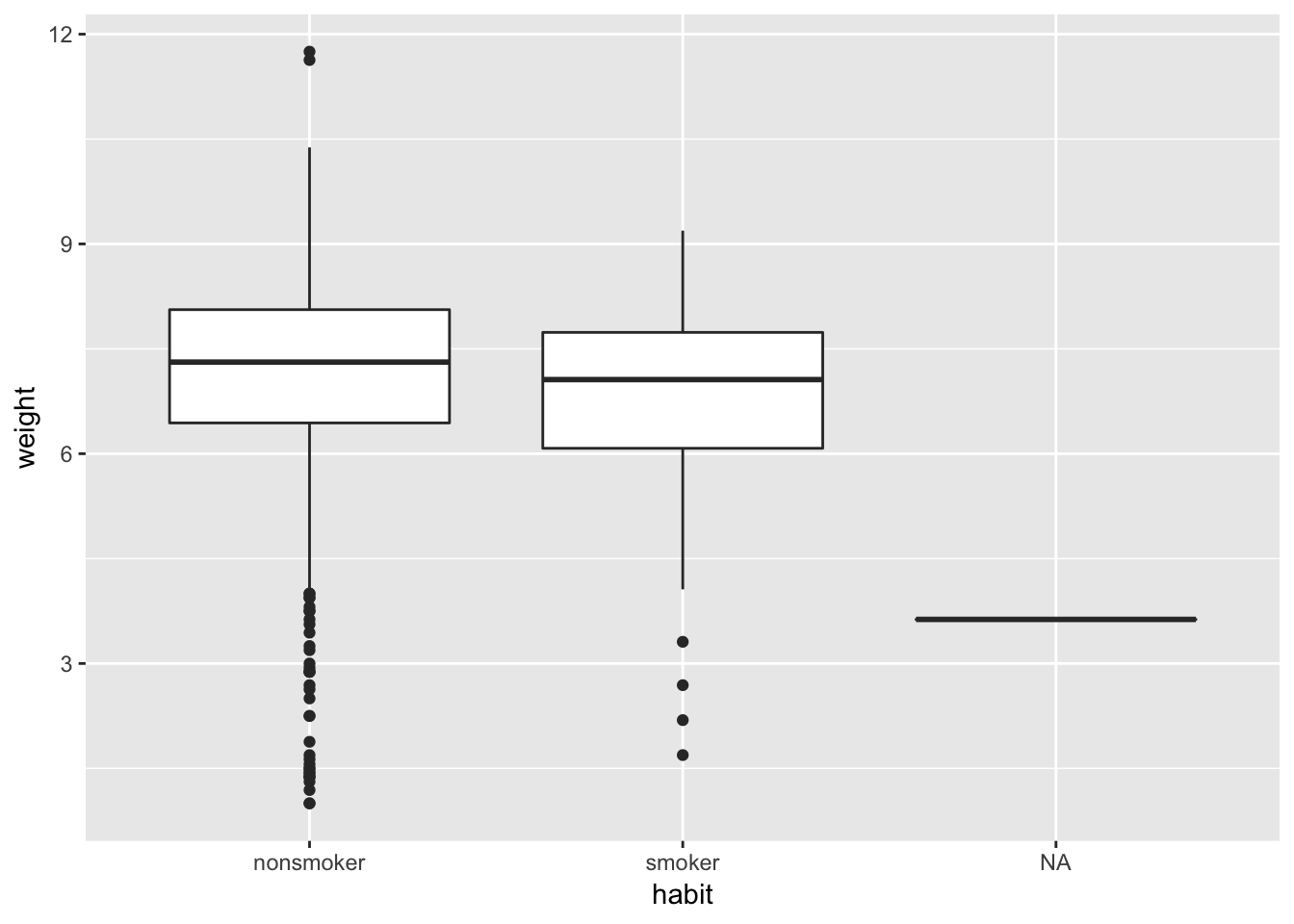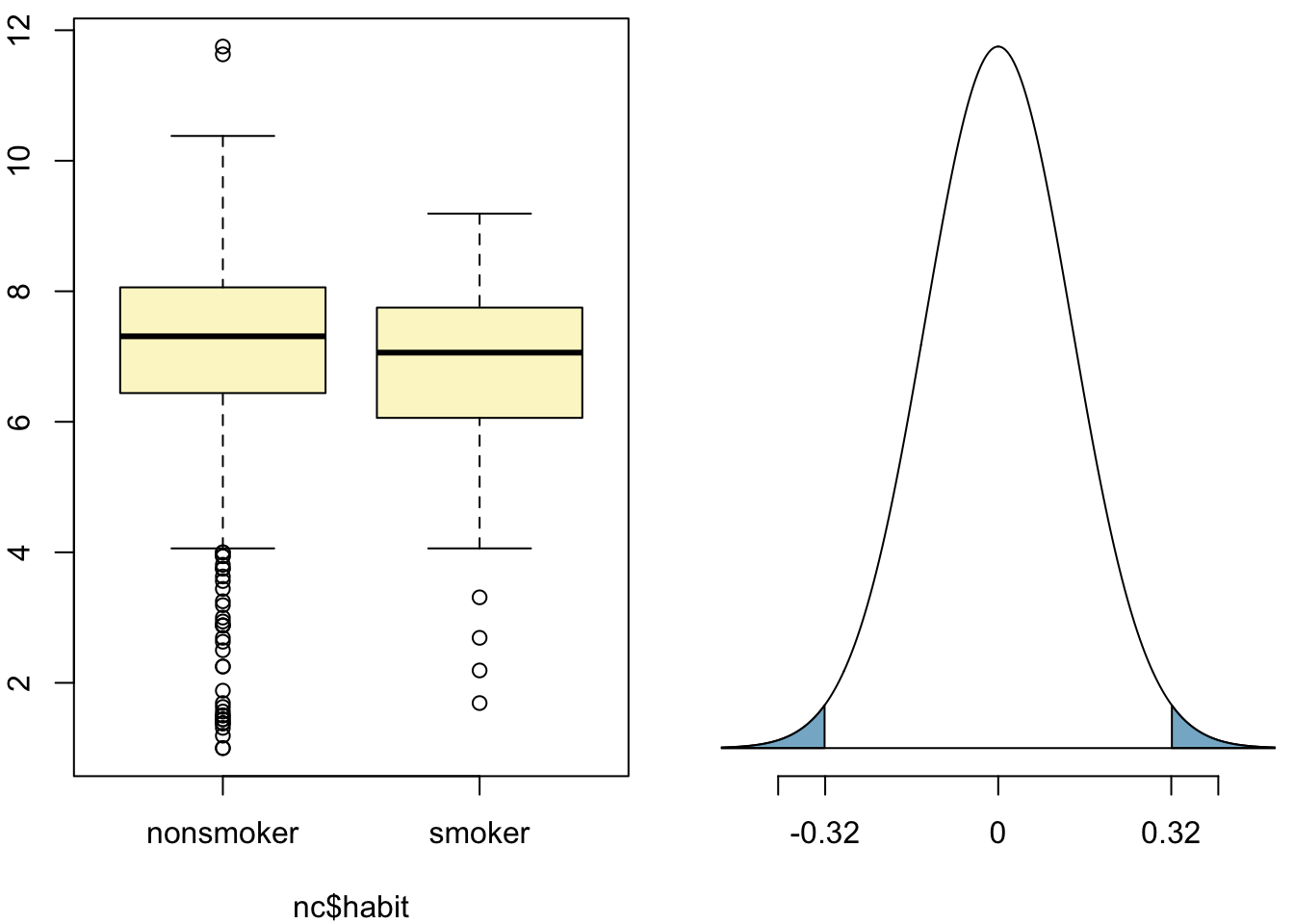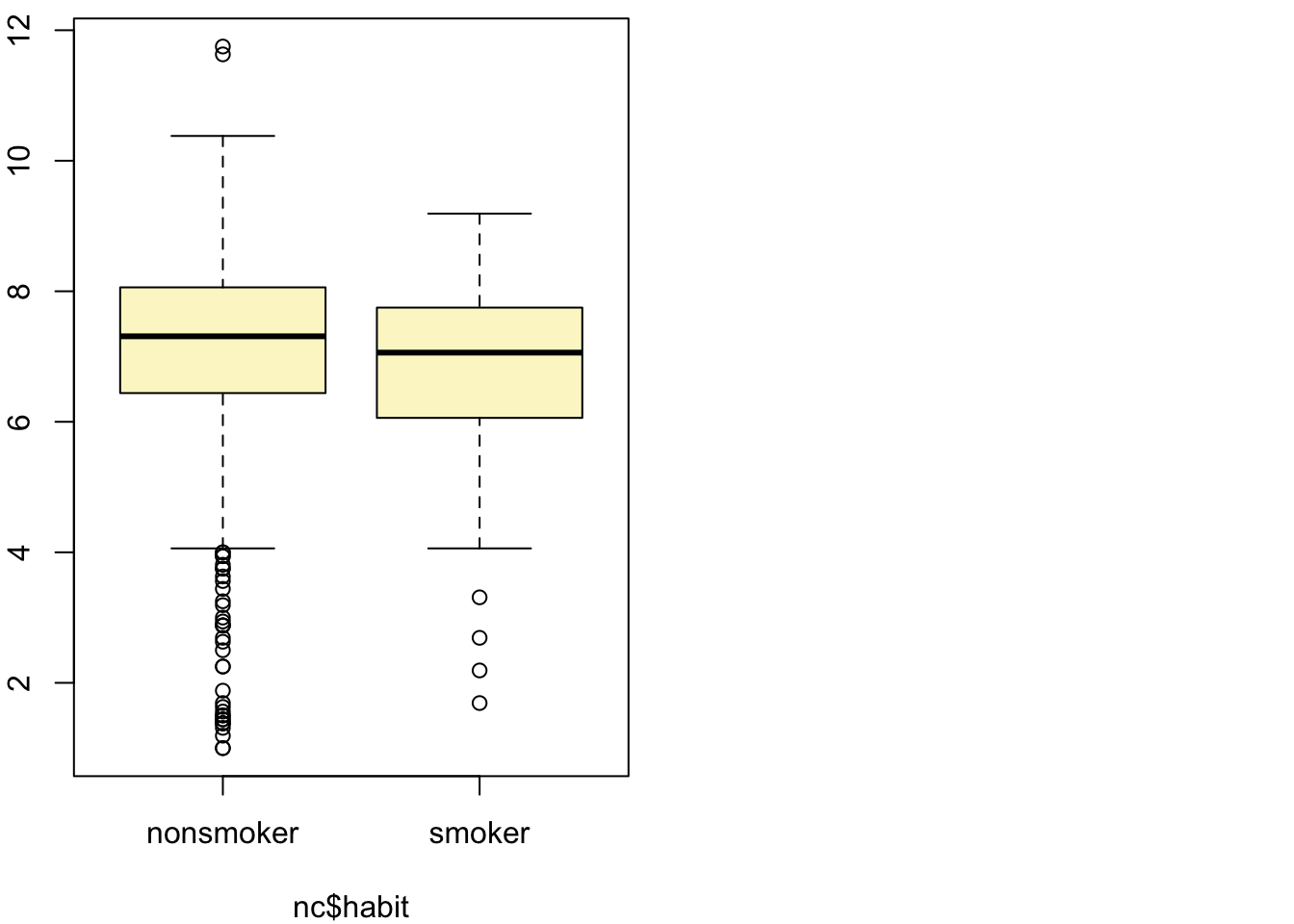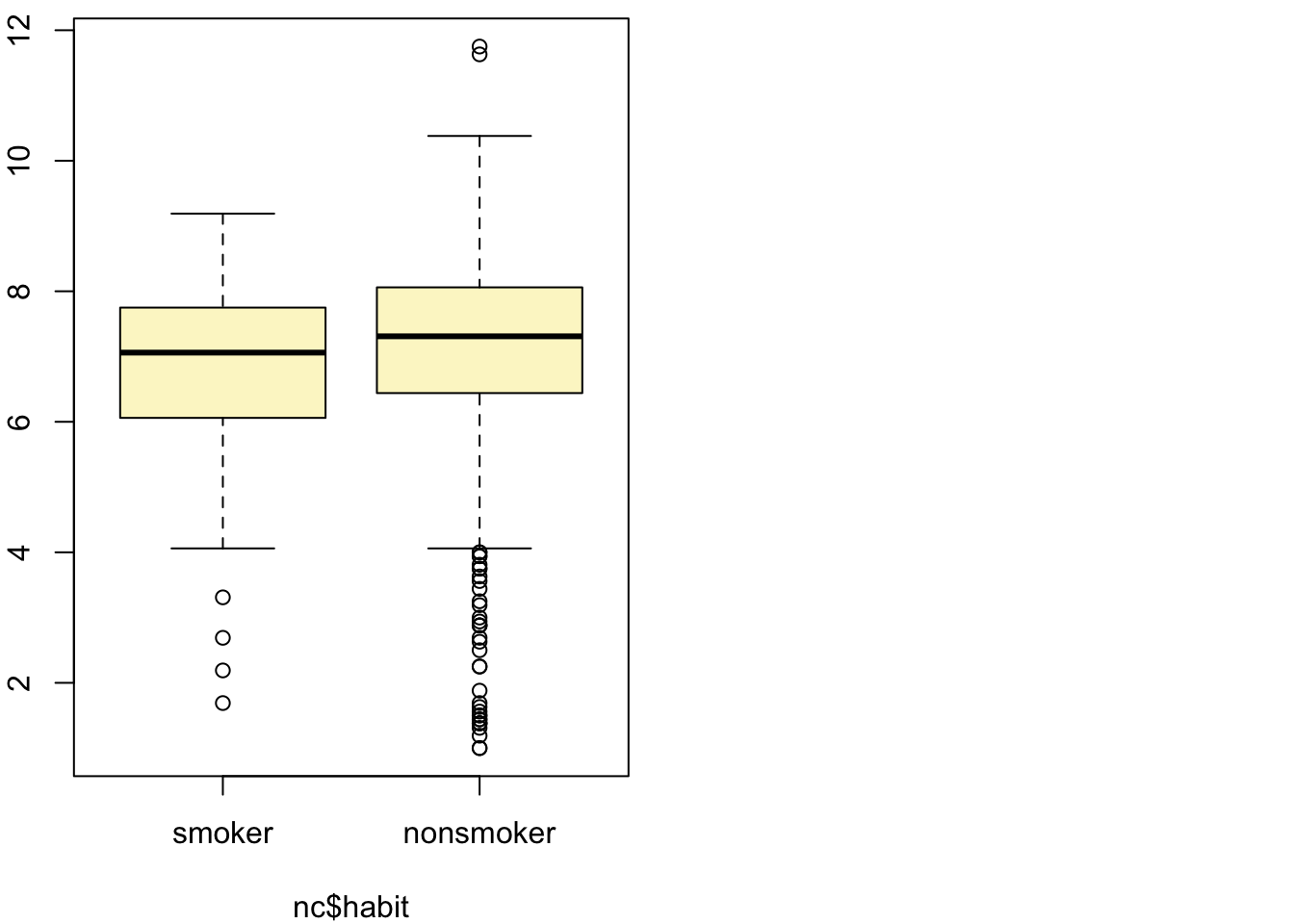## North Carolina births

In 2004, the state of North Carolina released a large data set containing information on births recorded in this state. This data set is useful to researchers studying the relation between habits and practices of expectant mothers and the birth of their children. We will work with a random sample of observations from this data set.

## Exploratory analysis

Load the nc data set into our workspace.

library(ggplot2)
## Warning: package 'ggplot2' was built under R version 3.4.4
load("more/nc.RData")

We have observations on 13 different variables, some categorical and some numerical. The meaning of each variable is as follows.

variable description
fage father’s age in years.
mage mother’s age in years.
mature maturity status of mother.
weeks length of pregnancy in weeks.
premie whether the birth was classified as premature (premie) or full-term.
visits number of hospital visits during pregnancy.
marital whether mother is married or not married at birth.
gained weight gained by mother during pregnancy in pounds.
weight weight of the baby at birth in pounds.
lowbirthweight whether baby was classified as low birthweight (low) or not (not low).
gender gender of the baby, female or male.
habit status of the mother as a nonsmoker or a smoker.
whitemom whether mom is white or not white.
1. What are the cases in this data set? How many cases are there in our sample?

The cases in this data set are births in the state of North Carolina. There are 1000 cases in the sample.

As a first step in the analysis, we should consider summaries of the data. This can be done using the summary command:

summary(nc)
##       fage            mage            mature        weeks
##  Min.   :14.00   Min.   :13   mature mom :133   Min.   :20.00
##  1st Qu.:25.00   1st Qu.:22   younger mom:867   1st Qu.:37.00
##  Median :30.00   Median :27                     Median :39.00
##  Mean   :30.26   Mean   :27                     Mean   :38.33
##  3rd Qu.:35.00   3rd Qu.:32                     3rd Qu.:40.00
##  Max.   :55.00   Max.   :50                     Max.   :45.00
##  NA's   :171                                    NA's   :2
##        premie        visits            marital        gained
##  full term:846   Min.   : 0.0   married    :386   Min.   : 0.00
##  premie   :152   1st Qu.:10.0   not married:613   1st Qu.:20.00
##  NA's     :  2   Median :12.0   NA's       :  1   Median :30.00
##                  Mean   :12.1                     Mean   :30.33
##                  3rd Qu.:15.0                     3rd Qu.:38.00
##                  Max.   :30.0                     Max.   :85.00
##                  NA's   :9                        NA's   :27
##      weight       lowbirthweight    gender          habit
##  Min.   : 1.000   low    :111    female:503   nonsmoker:873
##  1st Qu.: 6.380   not low:889    male  :497   smoker   :126
##  Median : 7.310                               NA's     :  1
##  Mean   : 7.101
##  3rd Qu.: 8.060
##  Max.   :11.750
##
##       whitemom
##  not white:284
##  white    :714
##  NA's     :  2
##
##
##
## 

As you review the variable summaries, consider which variables are categorical and which are numerical. For numerical variables, are there outliers? If you aren’t sure or want to take a closer look at the data, make a graph.

Consider the possible relationship between a mother’s smoking habit and the weight of her baby. Plotting the data is a useful first step because it helps us quickly visualize trends, identify strong associations, and develop research questions.

1. Make a side-by-side boxplot of habit and weight. What does the plot highlight about the relationship between these two variables?

The plot shows that the median weight of babies with mothers who are smokers is lower than the median weight of babies with mothers who are not smokers.

ggplot(data = nc, aes(x=habit, y=weight)) + geom_boxplot()The box plots show how the medians of the two distributions compare, but we can also compare the means of the distributions using the following function to split the weight variable into the habit groups, then take the mean of each using the mean function.

by(nc$weight, nc$habit, mean)
## nc$habit: nonsmoker ##  7.144273 ## -------------------------------------------------------- ## nc$habit: smoker
##  6.82873

There is an observed difference, but is this difference statistically significant? In order to answer this question we will conduct a hypothesis test.

## Inference

1. Check if the conditions necessary for inference are satisfied. Note that you will need to obtain sample sizes to check the conditions. You can compute the group size using the same by command above but replacing mean with length.

Random: The babies included in this dataset are selected randomly.

Normal: The sample size of each group is reasonably large (>30).

by(nc$weight, nc$habit, length)
## nc$habit: nonsmoker ##  873 ## -------------------------------------------------------- ## nc$habit: smoker
##  126

Independent: The birth statistics of each baby are independent (individual babies do not affect each other). Also, the sample size is less than 10% of the population.

1. Write the hypotheses for testing if the average weights of babies born to smoking and non-smoking mothers are different.

$$H_0$$: The mean weight of babies born to smoking mothers is equal to the mean weight of babies born to non-smoking mothers.

$$H_A$$: The mean weight of babies born to smoking mothers is not equal to the mean weight of babies born to non-smoking mothers.

Next, we introduce a new function, inference, that we will use for conducting hypothesis tests and constructing confidence intervals.

DATA606::inference(y = nc$weight, x = nc$habit, est = "mean", type = "ht", null = 0,
alternative = "twosided", method = "theoretical")
## Response variable: numerical, Explanatory variable: categorical
## Difference between two means
## Summary statistics:
## n_nonsmoker = 873, mean_nonsmoker = 7.1443, sd_nonsmoker = 1.5187
## n_smoker = 126, mean_smoker = 6.8287, sd_smoker = 1.3862
## Observed difference between means (nonsmoker-smoker) = 0.3155
##
## H0: mu_nonsmoker - mu_smoker = 0
## HA: mu_nonsmoker - mu_smoker != 0
## Standard error = 0.134
## Test statistic: Z =  2.359
## p-value =  0.0184Let’s pause for a moment to go through the arguments of this custom function. The first argument is y, which is the response variable that we are interested in: nc$weight. The second argument is the explanatory variable, x, which is the variable that splits the data into two groups, smokers and non-smokers: nc$habit. The third argument, est, is the parameter we’re interested in: "mean" (other options are "median", or "proportion".) Next we decide on the type of inference we want: a hypothesis test ("ht") or a confidence interval ("ci"). When performing a hypothesis test, we also need to supply the null value, which in this case is 0, since the null hypothesis sets the two population means equal to each other. The alternative hypothesis can be "less", "greater", or "twosided". Lastly, the method of inference can be "theoretical" or "simulation" based.

1. Change the type argument to "ci" to construct and record a confidence interval for the difference between the weights of babies born to smoking and non-smoking mothers.
DATA606::inference(y = nc$weight, x = nc$habit, est = "mean", type = "ci", null = 0,
alternative = "twosided", method = "theoretical")
## Response variable: numerical, Explanatory variable: categorical
## Difference between two means
## Summary statistics:
## n_nonsmoker = 873, mean_nonsmoker = 7.1443, sd_nonsmoker = 1.5187
## n_smoker = 126, mean_smoker = 6.8287, sd_smoker = 1.3862## Observed difference between means (nonsmoker-smoker) = 0.3155
##
## Standard error = 0.1338
## 95 % Confidence interval = ( 0.0534 , 0.5777 )

By default the function reports an interval for ($$\mu_{nonsmoker} - \mu_{smoker}$$). We can easily change this order by using the order argument:

inference(y = nc$weight, x = nc$habit, est = "mean", type = "ci", null = 0,
alternative = "twosided", method = "theoretical",
order = c("smoker","nonsmoker"))
## Response variable: numerical, Explanatory variable: categorical
## Difference between two means
## Summary statistics:
## n_smoker = 126, mean_smoker = 6.8287, sd_smoker = 1.3862
## n_nonsmoker = 873, mean_nonsmoker = 7.1443, sd_nonsmoker = 1.5187## Observed difference between means (smoker-nonsmoker) = -0.3155
##
## Standard error = 0.1338
## 95 % Confidence interval = ( -0.5777 , -0.0534 )

• Calculate a 95% confidence interval for the average length of pregnancies (weeks) and interpret it in context. Note that since you’re doing inference on a single population parameter, there is no explanatory variable, so you can omit the x variable from the function.
inference(y = nc\$weeks, est = "mean", type = "ci", null = 0,
alternative = "twosided", method = "theoretical")
## Single mean
## Summary statistics: